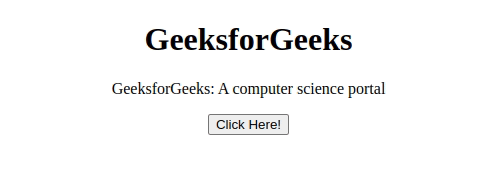GeeksforGeeks App
Open AppBrowser
Continue

# How to get character array from string in JavaScript ?

In this article, we will learn how to get a character array from a string in Javascript. The string is defined as the subsequence of characters. For getting the character we need to split out the characters.

The string in JavaScript can be converted into a character array by using:

JavaScript String split() Function: The str.split() function is used to split the given string into an array of strings by separating it into substrings using a specified separator provided in the argument.

Syntax:

`str.split(separator, limit)`

Example 1: In this example, we will get a character array from the string using the split() function in Javascript.

## HTML

 ```<``html``>`` ` `<``head``>``    ``<``title``>``        ``How to get character array from``        ``string in JavaScript?``    `````` ` `<``body` `style``=``"text-align:center;"``>``    ``<``h1` `style``=``"color:green"``>``        ``GeeksforGeeks``    ```` ` `    ``<``p` `id``=``"one"``>``        ``GeeksforGeeks: A computer science portal``    ```` ` `    ``<``input` `type``=``"button"``           ``value``=``"Click Here!"` `           ``onclick``=``"myGeeks()"``>`` ` `    ``<``script``>``        ``function myGeeks() {``            ``var str = document.getElementById("one").innerHTML;``            ``document.getElementById("one").innerHTML =``            ``str.split("");``        ``}``    `````` ` ``

Output:JavaScript Array.from() Function: The Array.from() function is an inbuilt function in JavaScript that creates a new array instance from a given array. In the case of a string, every alphabet of the string is converted to an element of the new array instance and in the case of integer values, a new array instance simply takes the elements of the given array.

Syntax:

`Array.from(str)`

Example 2: In this example, we will get a character array from the string using the Array.from() function in Javascript.

## HTML

 ```<``html``>`` ` `<``head``>``    ``<``title``>``        ``How to get character array from``        ``string in JavaScript?``    `````` ` `<``body` `style``=``"text-align:center;"``>``    ``<``h1` `style``=``"color:green"``>``        ``GeeksforGeeks``    ```` ` `    ``<``p` `id``=``"one"``>``        ``GeeksforGeeks: A computer science portal``    ```` ` `    ``<``input` `type``=``"button"` `           ``value``=``"Click Here!"` `           ``onclick``=``"myGeeks()"``>`` ` `    ``<``script``>``        ``function myGeeks() {``            ``var str = ``                ``document.getElementById("one").innerHTML;``            ``document.getElementById("one").innerHTML = ``            ``Array.from(str);``        ``}``    `````` ` ``

Output:JavaScript Spread Operator: Spread operator allows an iterable to expand in place. In the case of a string, it example string into character and we capture all the characters of a string in an array.

Syntax:

`var variableName = [ ...value ];`

Example3: In this example, we will get a character array from the string using the spread operator in Javascript.

## HTML

 ```<``html``>`` ` `<``head``>``    ``<``title``>``        ``How to get character array from``        ``string in JavaScript?``    `````` ` `<``body` `style``=``"text-align:center;"``>`` ` `    ``<``h1` `style``=``"color:green"``>``        ``GeeksforGeeks``    ```` ` `    ``<``p` `id``=``"one"``>``        ``GeeksforGeeks: A computer science portal``    ```` ` `    ``<``input` `type``=``"button"` `           ``value``=``"Click Here!"` `           ``onclick``=``"myGeeks()"``>`` ` `    ``<``script``>``        ``function myGeeks() {``            ``var str = ``                ``document.getElementById("one").innerHTML;``            ``document.getElementById("one").innerHTML = ``             ``[...str];``        ``}``    `````` ` ``

Output:JavaScript is best known for web page development but it is also used in a variety of non-browser environments. You can learn JavaScript from the ground up by following this JavaScript Tutorial and JavaScript Examples.

My Personal Notes arrow_drop_up
Related Tutorials